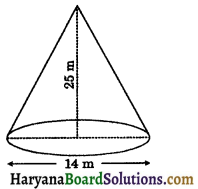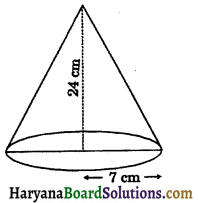# HBSE 9th Class Maths Solutions Chapter 13 Surface Areas and Volumes Ex 13.3

Haryana State Board HBSE 9th Class Maths Solutions Chapter 13 Surface Areas and Volumes Ex 13.3 Textbook Exercise Questions and Answers.

## Haryana Board 9th Class Maths Solutions Chapter 13 Surface Areas and Volumes Exercise 13.3

Assume π = $$\frac{22}{7}$$, unless stated otherwise:

Question 1.
Diameter of the base of a cone is 10.5 cm and its slant height is 10 cm. Find its curved surface area.
Solution:
We have,
Slant height of the cone (l) = 10 cm
and diameter of the base of a cone = 10.5 cm
∴ Radius of the base of a cone (r) = $$\frac{22}{7}$$
= 5.25 cm
∴ Curved surface area of the cone = πrl
= $$\frac{22}{7}$$ × 5.25 × 10
= 165 cm2
Hence, curved surface area of the cone = 165 cm2

Question 2.
Find the total surface area of a cone, if its slant height is 21 m and diameter of its base is 24 m.
Solution:
We have,
Slant height of a cone (l) = 21 m
and diameter of base of a cone = 24 m
∴ Radius of base of a cone (r) = $$\frac{24}{2}$$ = 12 m
∴ Total surface area of a cone (r) = πr(l + r)
= $$\frac{22}{7}$$ × 12(21 + 12)
= $$\frac{22}{7}$$ × 12 × 33
= 1244.57 m2 (approx.).
Hence, total surface area of a cone = 1244.57 m2 (approx.).Question 3.
Curved surface area of a cone is 308 cm2 and its slant height is 14 cm. Find : (i) radius of the base and (ii) total surface area of the cone.
Solution:
We have,
Slant height of a cone (l) = 14 cm
Let radius of the base of a cone be r сm.
(i) curved surface area of a cone = 308 cm2
⇒ πrl = 308
⇒ $$\frac{22}{7}$$ × r × 14 = 308
⇒ 44r = 308
⇒ r = $$\frac{308}{44}$$
⇒ r = 7 cm.

(ii) Total surface area of the cone = πr(l + r)
= $$\frac{22}{7}$$ × 7(14 + 7)
= 22 × 21
= 462 cm2
Hence, (i) radius of the base of a cone = 7 cm
(ii) total surface area of a cone = 462 cm2.

Question 4.
A conical tent is 10 m high and the radius of its base is 24 m. Find :
(i) slant height of the tent.
(ii) cost of the canvas required to make the tent, if the cost of 1 m2 canvas is Rs. 70.
Solution:
We have,
Height of the conical tent (h) = 10 m
and radius of the base of conical tent (r) = 24 m
(i) Let slant height of the cone be l m.
∴ l = $$\sqrt{h^2+r^2}$$
⇒ l = $$\sqrt{10^2+24^2}$$
⇒ l = $$\sqrt{100+576}$$
⇒ l = $$\sqrt{676}$$
⇒ l = 26 m
(ii) Required area of canvas to make the conical tent = Curved surface area of the cone
= πrl = $$\frac{22}{7}$$ × 24 × 26
= $$\frac{13728}{7}$$ m2
∵ 1 m2 canvas cost = Rs. 70
∴ $$\frac{13728}{7}$$ m2 canvas cost = Rs. $$\frac{70 \times 13728}{7}$$
= Rs. 137280
Hence, (i) Slant height of the tent = 26 m
(ii) Cost of canvas = Rs. 137280.Question 5.
What length of tarpaulin 3 m wide will be required to make conical tent of height 8 m and base radius 6 m? Assume that the extra length of material that will be required for stitching margins and wastage in cutting is approximately 20 cm (Use π = 3.14)
Solution:
We have, Height of the conical tent (h) = 8 m
Radius of base of conical tent (r) = 6 m
∴ l = $$\sqrt{h^2+r^2}$$
⇒ l = $$\sqrt{8^2+6^2}$$
⇒ l = $$\sqrt{64+36}$$
⇒ l = $$\sqrt{100}$$
⇒ l = 10 m
Area of tarpaulin required to make the conical tent = Curved surface area of the cone
= πrl = 3.14 × 6 × 10
= 188.4 m2
∵ Width of tarpaulin = 3 m.
∴ Length of tarpaulin = $$\frac{\text { Area of tarpaulin }}{\text { Width of tarpaulin }}$$
$$\frac{188\cdot4}{3}$$ = 62.8 m
Wastage in cutting = 20 cm = 0.20 m
Total length of tarpaulin = 62.8 + 0.20
= 63 m
Hence,length of tarpualin = 63 m.

Question 6.
The slant height and base diameter of a conical tomb are 25 m and 14 m respectively. Find the cost of whitewashing its curved surface at the rate of Rs. 210 per 100 m2
Solution:
We have,
Slant height of a conical tomb (l) = 25 m
and diameter of a conical tomb = 14 m
∴ Radius of a conical tomb (r) = $$\frac{14}{2}$$ = 7m∴ Curved surface area of the conical tomb = πrl
= $$\frac{22}{7}$$ × 7 × 25
= 550 m2
∵ Cost of white washing of 100 m2 of tomb
= Rs. 210
∴ Cost of white washing of 550 m2 of tomb
= Rs $$\frac{210 \times 550}{100}$$
= Rs. 1155
Hence,cost of white washing of tomb = Rs. 1155.

Question 7.
A joker’s cap is in the form of a right circular cone of base radius 7 cm and height 24 cm. Find the area of the sheet required to make 10 such caps.
Solution:
We have,
Radius of conical cap (r) = 7 cm
and height of the conical cap (h) = 24 cm∴ l = $$\sqrt{h^2+r^2}$$
⇒ l = $$\sqrt{24^2+7^2}$$
⇒ l = $$\sqrt{576+49}$$
⇒ l = $$\sqrt{625}$$
⇒ l = ±25
⇒ l = 25
∴ Curved surface area of a cap = πrl
= $$\frac{22}{7}$$ × 7 × 25 = 550 cm2
Curved surface area of 10 such caps = 550 × 10 = 5500 cm2
Hence, required area of sheet to make 10 such caps = 5500 cm2.Question 8.
A bus stop is barricaded from the remaining part of the road, by using 50 hollow cones made of recycled cardboard, Each cone has a base diameter of 40 cm and height 1 m. If the outer side of each of the cone is to be painted and the cost of painting is Rs. 12 per m2, what will be the cost of painting all these cones? (Use π = 3.14 and take $$\sqrt{1.04}$$ = 1.02)
Solution:
We have,
Height of a cone (h) = 1 m = 100 cm
and diameter of a cone (d) = 40 cm
∴ Radius of a cone (r) = $$\frac{40}{2}$$ = 20 cm
∴ l = $$\sqrt{h^2+r^2}$$
⇒ l = $$\sqrt{100^2+20^2}$$
⇒ l = $$\sqrt{10000+400}$$
⇒ l = $$\sqrt{10400}$$
⇒ l = 101.98
⇒ l = 102 (approx.)
Outer surface area of 1 cone = πrl = 3.14 × 20 × 102 = 6405.6 cm2
Outer surface area of 50 cones= 6405.6 × 50 = 320280 cm2
$$\frac{320280}{10000}$$ m2
= 32.028 m2
Cost of painting of 1 m2 = Rs. 12
Cost of painting of 32.028 m2 = Rs. 12 × 32.028
= Rs. 384.336
= Rs. 384.34
Hence, cost of painting of 50 cones = Rs. 384.34.